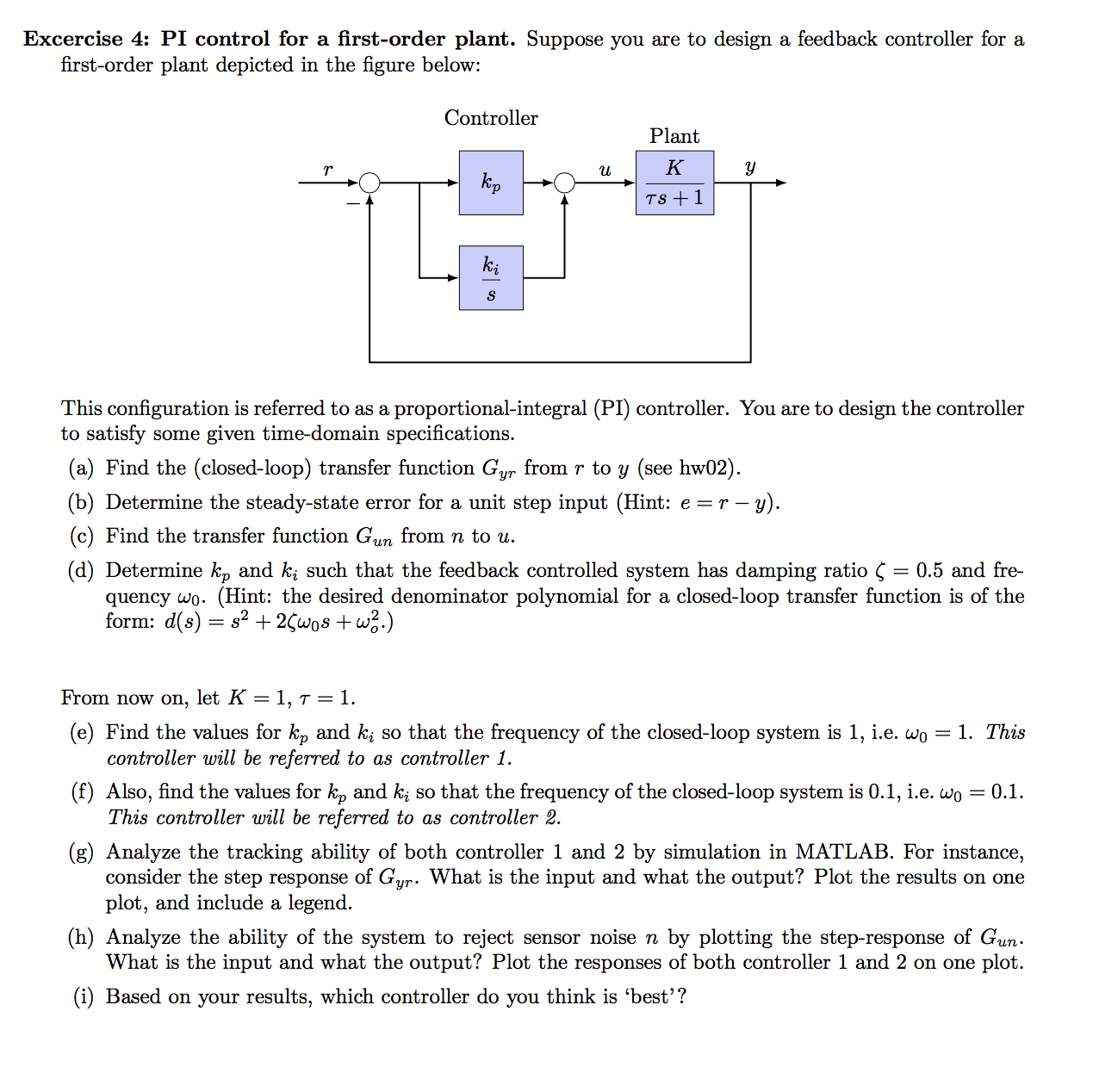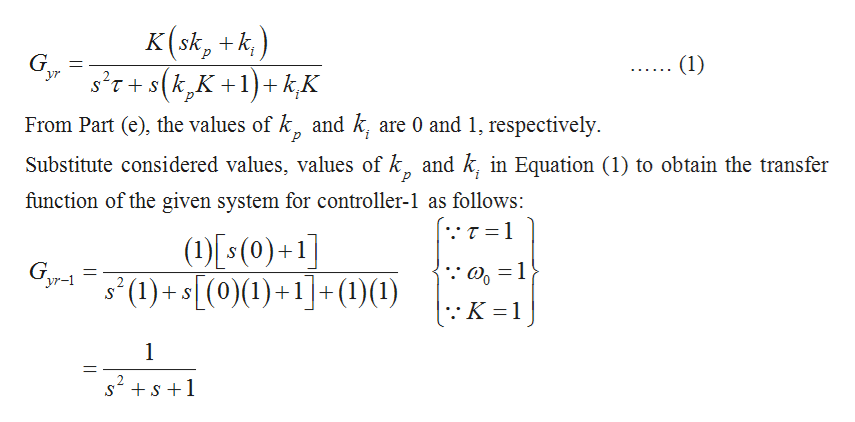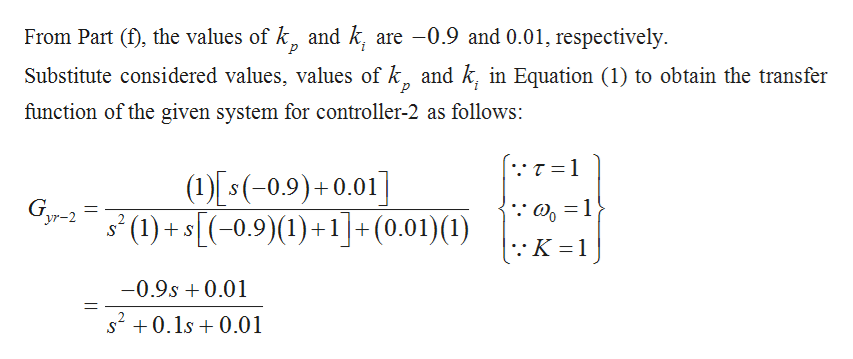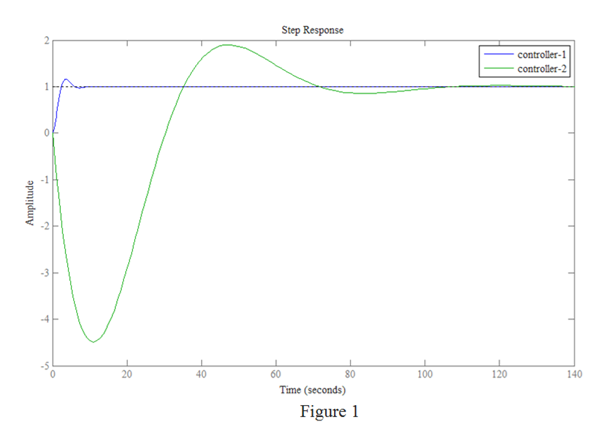# Excercise 4: PI control for a first-order plant. Suppose you are to design a feedback controller for afirst-order plant depicted in the figure below:ControllerPlantКkpTS 1SThis configuration is referred to as a proportional-integral (PI) controller. You are to design the controllerto satisfy some given time-domain specifications.(a) Find the (closed-loop) transfer function Gyr from r to y (see hw02).(b) Determine the steady-state error for a unit step input (Hint: e r --y)(c) Find the transfer function Gun from n to u.(d) Determine k, and ki such that the feedback controlled system has damping ratio Ç = 0.5 and fre-quency wo. (Hint: the desired denominator polynomial for a closed-loop transfer function is of theform: d(s) s2+ 2Çwos +w2.)From now on, let K = 1, t = 1.(e) Find the values for kp and ki so that the frequency of the closed-loop system is 1, i.e. wo = 1. Thiscontroller will be referred to as controller 1.(f) Also, find the values for k, and k so that the frequency of the closed-loop system is 0.1, i.e. woThis controller will be referred to as controller 2.= 0.1(g) Analyze the tracking ability of both controller 1 and 2 by simulation in MATLAB. For instance,consider the step response of Gur. What is the input and what the output? Plot the results on oneplot, and include a legend(h) Analyze the ability of the system to reject sensor noise n by plotting the step-response of GunWhat is the input and what the output? Plot the responses of both controller 1 and 2 on one plot.(i) Based on your results, which controller do you think is 'best'?

Questionhelp_outlineImage TranscriptioncloseExcercise 4: PI control for a first-order plant. Suppose you are to design a feedback controller for a first-order plant depicted in the figure below: Controller Plant К kp TS 1 S This configuration is referred to as a proportional-integral (PI) controller. You are to design the controller to satisfy some given time-domain specifications. (a) Find the (closed-loop) transfer function Gyr from r to y (see hw02). (b) Determine the steady-state error for a unit step input (Hint: e r - -y) (c) Find the transfer function Gun from n to u. (d) Determine k, and ki such that the feedback controlled system has damping ratio Ç = 0.5 and fre- quency wo. (Hint: the desired denominator polynomial for a closed-loop transfer function is of the form: d(s) s2+ 2Çwos +w2.) From now on, let K = 1, t = 1. (e) Find the values for kp and ki so that the frequency of the closed-loop system is 1, i.e. wo = 1. This controller will be referred to as controller 1. (f) Also, find the values for k, and k so that the frequency of the closed-loop system is 0.1, i.e. wo This controller will be referred to as controller 2. = 0.1 (g) Analyze the tracking ability of both controller 1 and 2 by simulation in MATLAB. For instance, consider the step response of Gur. What is the input and what the output? Plot the results on one plot, and include a legend (h) Analyze the ability of the system to reject sensor noise n by plotting the step-response of Gun What is the input and what the output? Plot the responses of both controller 1 and 2 on one plot. (i) Based on your results, which controller do you think is 'best'? fullscreen
check_circle

Step 1

Part (g):

From Part (a), the closed-loop transfer function of the given system is obtained as follows:help_outlineImage Transcriptionclose) K (sk, k (1) s'T+S(k,K+1)kK yr From Part (e), the values of k, and k, are 0 and 1, respectively Substitute considered values, values of k and k, in Equation (1) to obtain the transfer function of the given system for controller-1 as follows: .τ=1 (1s(0)+1] (1)+(0)(1)+1(1)(1) 1 1 fullscreen
Step 2help_outlineImage TranscriptioncloseFrom Part (f), the values of k, and k, are -0.9 and 0.01, respectively р Substitute considered values, values of k and k, in Equation (1) to obtain the transfer function of the given system for controller-2 as follows: T 1 (s(-0,9)+0.01] (1+5-0.9)(1)+1+(0.01)(1) 'yr-2 S 11 -0.9s 0.01 s20.1s0.01 fullscreen
Step 3

From the obtained transfer functions, write the MATLAB code for the step-response of the given system for controller-1 and controller-2 as follows:

num1=;

den1=[1 1 1];

y1=tf(num1,den1);

num2=[-0.9 0.01];

den2=[1 0....help_outlineImage TranscriptioncloseStep Response controller-1 controller-2 3 20 40 60 80 100 120 140 Time (seconds) Figure 1 Amplitude fullscreen

### Want to see the full answer?

See Solution

#### Want to see this answer and more?

Solutions are written by subject experts who are available 24/7. Questions are typically answered within 1 hour.*

See Solution
*Response times may vary by subject and question.
Tagged in

### Electrical Engineering